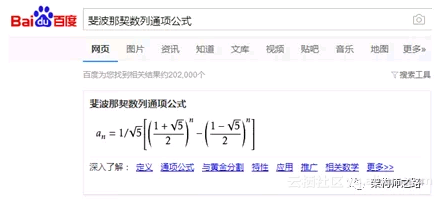#0
5
01. 云栖社区>
2. 架构师之路>
3. 博客>
4. 正文

## 拜托，面试别再问我斐波那契数列了！！！

f(0) = 0;

f(1) = 1;

f(n) = f(n-1) + f(n-2)  (当n>=2时)

uint32_t f(uint32_t n){

if(n==0) return 0;

if(n==1) return 1;

return f(n-1)+f(n-2);

}

f(5) = f(4) + f(3);

f(4) = f(3)+ f(2);

static uint32_t count=0; // 加一个全局变量计数

uint32_t f(uint32_t n){

count++;  // 递归一次，计数加一

if(n==0) return 0;

if(n==1) return 1;

return f(n-1)+f(n-2);

}

f(5) count = 15

f(10) count = 177

f(15) count = 1K+

f(20) count = 2W+

f(25) count = 24W+

f(30) count = 269W+

f(35) count = 2986W+

f(40) count = 3.3Y+

f(45) … 抱歉，我机器太慢，算不出来

（1）这个count，是函数递归了对少次；

（2）f(45)，机器居然算不出来；

（3）对结论有疑问的，自己可以run一把；

（1）斐波那契数列求解，如果用直接法，时间复杂度是指数级的，不可行；

（2）如果没有太大的把握，工程中尽量少使用递归，容易把自己玩死；

f(0) = 0;

f(1) = 1;

f(n) = f(n-1) + f(n-2) n>=2时

uint32_t f(uint32_t n){

uint32_t arr[n];

arr=0;

arr=1;

for(uint32_t i=2;i<=n;i++){

arr[i]=arr[i-1]+arr[i-2];

}

return arr[n];

}

f(0) = 0;

f(1) = 1;

f(n) = f(n-1) + f(n-2)  (当n>=2时)

f(n) = f(n-1) + f(n-2)

x^2 = x + 1

x1=(1+√5)/2

x2=(1-√5)/2

f(n) = a1(x1)^n + a2(x2)^n

f(0) = 0;

f(1) = 1;

a1=1/√5

a2=-1/√5

f(n)=(1/√5)*{[(1+√5)/2]^n -[(1-√5)/2]^n}f(n) = a1(b1)^n + a2(b2)^n

uint64_t power(uint64_t a, uint64_t n){

uint64_t result=a;

for(uint64_t i=1;i<n;i++){

result *=a;

}

return result;

}

a^n减治法思路

uint64_t power(uint64_t a, uint64_t n){

if(n==0)return 1;

if(n==1)return a;

uint64_t r=0;

if(n%2){

r=power(a, (n-1)/2);

return r*r*a;

}

else{

r=power(a, n/2);

return r*r;

}

}

result=0;

result=1;

result=1;

result=2;

result=3;

return result[n];

+ 关注